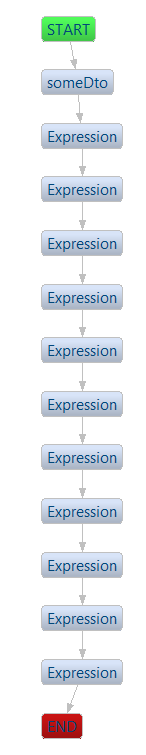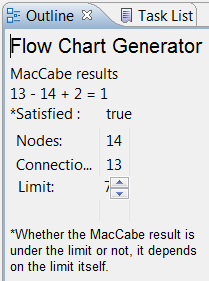# How is the cyclomatic complexity of an algorithm calculated ?

When checking the issues detected by Sonar, i noticed a few issues related to cyclomatic complexity.
The usual message is clear :

The Cyclomatic Complexity of this method “checkSomething” is 22 which is greater than 12 authorized.

Indeed, the method checkSomething has way too many if conditions :

```private static SomeDto checkSomething(AnotherDto anotherDto, String reference)
{
SomeDto someDto = new SomeDto();

// condition 1
if (!someDto.getA())
return new SomeDto("bla1", "blabla");

// condition 2
if (someDto.getName2() == null || checkSurName(anotherDto.getName()))
return new SomeDto("bla2", "blabla");

// condition 3
if (someDto.getName3() == null || checkSurName(anotherDto.getName()))
return new SomeDto("bla3", "blabla");

// condition 4
if (someDto.getName4() == null && checkSurName(anotherDto.getName()))
return new SomeDto("bla4", "blabla");

// condition 5
if (someDto.getName5() == null || checkSurName(anotherDto.getName()))
return new SomeDto("bla5", "blabla");

// condition 6
if (someDto.getName6() == null && checkSurName(anotherDto.getName()))
return new SomeDto("bla6", "blabla");

// condition 7
if (someDto.getName7() == null && checkSurName(anotherDto.getName()))
return new SomeDto("bla7", "blabla");

// condition 8
if (someDto.getName8() == null && checkSurName(anotherDto.getName()))
return new SomeDto("bla8", "blabla");

// condition 9
if (someDto.getName9() == null && checkSurName(anotherDto.getName()))
return new SomeDto("bla9", "blabla");

// condition 10
if (someDto.getName10() == null && checkSurName(anotherDto.getName()))
return new SomeDto("bla10", "blabla");

// condition 11
if (someDto.getName11() == null && checkSurName(anotherDto.getName()))
return new SomeDto("bla11", "blabla");

return someDto;
}
```

Question : how is the cyclomatic complexity of an algorithm calculated ?
Cyclomatic complexity is the number of independent paths through the program.
A program can be seen as a connected graph.
The formula is the following :
v(g) = e – n + 2P
where
e = number of edges
n = number of nodes
P = the number of exit points (usually only 1)

An edge connects 2 nodes. It represents non-branching and branching links between nodes.
Nodes are decisions points which may be conditional statements like if, if … else, switch, while, etc.
This metric was developed by Thomas J. McCabe in 1976.

Well, after further investigation and according to this link (checkstyle tool), I have concluded that the McCabe formula is not really applied to calculate the cyclomatic complexity in a Java program.

The complexity is equal to the number of decision points + 1 Decision points: if, while , do, for, ?:, catch , switch, case statements, and operators && and || in the body of target.

So if I apply this rule to the previous sample code :

```
private static SomeDto checkSomething(AnotherDto anotherDto, String reference)  // 1
{
SomeDto someDto = new SomeDto();

// condition 1
if (!someDto.getA())                                 // 2
return new SomeDto("bla1", "blabla");

// condition 2
if (someDto.getName2() == null || checkSurName(anotherDto.getName()))  // 4
return new SomeDto("bla2", "blabla");

// condition 3
if (someDto.getName3() == null || checkSurName(anotherDto.getName()))  // 6
return new SomeDto("bla3", "blabla");

// condition 4
if (someDto.getName4() == null && checkSurName(anotherDto.getName()))  // 8
return new SomeDto("bla4", "blabla");

// condition 5
if (someDto.getName5() == null || checkSurName(anotherDto.getName()))  // 10
return new SomeDto("bla5", "blabla");

// condition 6
if (someDto.getName6() == null && checkSurName(anotherDto.getName())) // 12
return new SomeDto("bla6", "blabla");

// condition 7
if (someDto.getName7() == null && checkSurName(anotherDto.getName()))  // 14
return new SomeDto("bla7", "blabla");

// condition 8
if (someDto.getName8() == null && checkSurName(anotherDto.getName()))  // 16
return new SomeDto("bla8", "blabla");

// condition 9
if (someDto.getName9() == null && checkSurName(anotherDto.getName()))  // 18
return new SomeDto("bla9", "blabla");

// condition 10
if (someDto.getName10() == null && checkSurName(anotherDto.getName())) // 20
return new SomeDto("bla10", "blabla");

// condition 11
if (someDto.getName11() == null && checkSurName(anotherDto.getName())) // 22
return new SomeDto("bla11", "blabla");

return someDto;
}
```

Anyways, the result number of 22 makes sense.
This method has an awful number of successive if conditions and something should be done about it to improve its maintainability. If we would apply strictly the McCabe formula, the result would be strange. At least according to this plugin for Eclipse : Eclipse Control Flow Graph Generator
The flow chart generated is the following :And the McCabe result would be 1 !| 750 Words